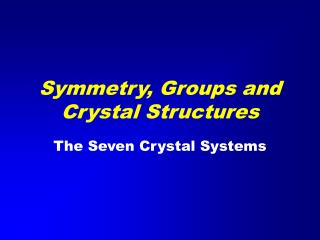Download PresentationSymmetry, Groups and Crystal Structures

# Symmetry, Groups and Crystal Structures

Download Presentation## Symmetry, Groups and Crystal Structures

- - - - - - - - - - - - - - - - - - - - - - - - - - - E N D - - - - - - - - - - - - - - - - - - - - - - - - - - -
##### Presentation Transcript

1. Symmetry, Groups and Crystal Structures The Seven Crystal Systems

2. Minerals structures are described in terms of the unit cell

3. The Unit Cell • The unit cell of a mineral is the smallest divisible unit of mineral that possesses the symmetry and properties of the mineral. • It is a small group of atoms arranged in a “box” with parallel sides that is repeated in three dimensions to fill space. • It has three principal axes (a, b and c) and • Three interaxial angles (a, b, and g)

4. The Unit Cell • a is angle between b and c • b is angle between a and c • g is angle between a and b

5. Seven Crystal Systems • The presence of symmetry operators places constraints on the geometry of the unit cell. • The different constraints generate the seven crystal systems. • Triclinic Monoclinic • Orthorhombic Tetragonal • Trigonal Hexagonal • Cubic (Isometric)

6. Seven Crystal Systems • Triclinic a b  c; a b  g  90º 120º • Monoclinic a b  c; a=g = 90º; b  90º 120º • Orthorhombic a b  c; a=b =g = 90º • Tetragonala= b  c; a=b =g = 90º • Trigonal a= b  c; a=b =90º; g =120º • Hexagonal a=b  c; a=b =90º; g =120º • Cubic a=b=c; a=b =g = 90º

7. Symmetry Operations • A symmetry operation is a transposition of an object that leaves the object invariant. • Rotations • 360º, 180º, 120º, 90º, 60º • Inversions (Roto-Inversions) • 360º, 180º, 120º, 90º, 60º • Translations: • Unit cell axes and fraction thereof. • Combinations of the above.

8. Rotations • 1-fold 360º I Identity • 2-fold 180º 2 • 3-fold 120º 3 • 4-fold 90º 4 • 6-fold 60º 6

9. Roto-Inversions(Improper Rotations) • 1-fold 360º • 2-fold 180º • 3-fold 120º • 4-fold 90º • 6-fold 60º

10. Translations • Unit Cell Vectors • Fractions of unit cell vectors • (1/2, 1/3, 1/4, 1/6) • Vector Combinations

11. Groups • A set of elements form a group if the following properties hold: • Closure: Combining any two elements gives a third element • Association: For any three elements (ab)c = a(bc). • Identity: There is an element, I such that Ia = aI = a • Inverses: For each element, a, there is another element b such that ab = I = ba

12. Groups • The elements of our groups are symmetry operators. • The rules limit the number of groups that are valid combinations of symmetry operators. • The order of the group is the number of elements.

13. Point Groups (Crystal Classes) • We can do symmetry operations in two dimensions or three dimensions. • We can include or exclude the translation operations. • Combining proper and improper rotation gives the point groups (Crystal Classes) • 32 possible combinations in 3 dimensions • 32 Crystal Classes (Point Groups) • Each belongs to one of the (seven) Crystal Systems

14. Space Groups • Including the translation operations gives the space groups. • 17 two-dimensional space groups • 230 three dimensional space groups • Each space group belongs to one of the 32 Crystal Classes (remove translations)

15. Crystal Morphology • A face is designated by Miller indices in parentheses, e.g. (100) (111) etc. • A form is a face plus its symmetric equivalents (in curly brackets) e.g {100}, {111}. • A direction in crystal space is given in square brackets e.g. , .

16. Halite Cube

17. Miller Indices • Plane cuts axes at intercepts (,3,2). • To get Miller indices, invert and clear fractions. • (1/, 1/3, 1/2) (x6)= • (0, 2, 3) • General face is (h,k,l)

18. Miller Indices • The cube face is (100) • The cube form {100} is comprises faces (100),(010),(001), (-100),(0-10),(00-1)

19. Halite Cube (100)

20. Stereographic Projections • Used to display crystal morphology. • X for upper hemisphere. • O for lower.

21. Stereographic Projections • We will use stereographic projections to plot the perpendicular to a general face and its symmetry equivalents (general form hkl). • Illustrated above are the stereographic projections for Triclinic point groups 1 and -1.